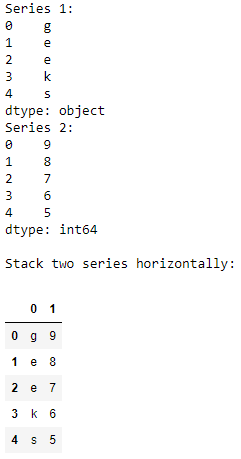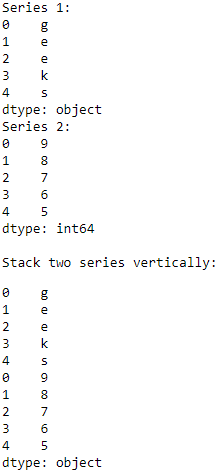# Stack two Pandas series vertically and horizontally

• Last Updated : 18 Aug, 2020

In this article we’ll see how we can stack two Pandas series both vertically and horizontally. We stack these lists to combine some data in a DataFrame for a better visualization of the data, combining different data, etc. Now let’s see with the help of examples how we can do this.

Stacking Horizontally : We can stack 2 Pandas series horizontally by passing them in the pandas.concat() with the parameter `axis = 1`.
Example :

 `# importing the module ` `import` `pandas as pd ` ` `  `# creating the series ` `series1 ``=` `pd.Series([``'g'``, ``'e'``, ``'e'``, ``'k'``, ``'s'``]) ` `print``(``"Series 1:"``) ` `print``(series1) ` `series2 ``=` `pd.Series([``9``, ``8``, ``7``, ``6``, ``5``]) ` `print``(``"Series 2:"``) ` `print``(series2) ` `  `  `# stacking the series horizontally ` `df ``=` `pd.concat([series1, series2], axis ``=` `1``) ` `print``(``"\nStack two series horizontally:"``) ` `display(df) `

Output :Stacking Vertically : We can stack 2 Pandas series vertically by passing them in the `pandas.concat()` with the parameter `axis = 0`.
Example :

 `# importing the module ` `import` `pandas as pd ` ` `  `# creating the series ` `series1 ``=` `pd.Series([``'g'``, ``'e'``, ``'e'``, ``'k'``, ``'s'``]) ` `print``(``"Series 1:"``) ` `print``(series1) ` `series2 ``=` `pd.Series([``9``, ``8``, ``7``, ``6``, ``5``]) ` `print``(``"Series 2:"``) ` `print``(series2) ` `  `  `# stacking the series vertically ` `df ``=` `pd.concat([series1, series2], axis ``=` `0``) ` `print``(``"\nStack two series vertically:"``) ` `display(df) `

Output :My Personal Notes arrow_drop_up
Recommended Articles
Page :